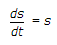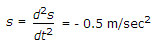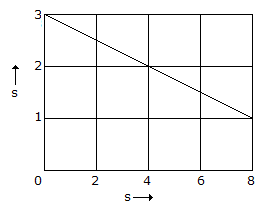# Civil Engineering - UPSC Civil Service Exam Questions

26.

For the motion represented by the graph shown in the given figure, the numerical value of velocity, when accelerationwill beA. 1 m/sec B. 2 m/sec C. 3 m/sec D. 4 m/sec

Explanation:

No answer description available for this question. Let us discuss.

27.

A star is observed at its upper culmination when it is north of zenith. The latitude of the place of observation is 30°N and declination of the star is 50°N. The zenith distance of the star is

 A. 10° B. 20° C. 40° D. 80°

Explanation:

No answer description available for this question. Let us discuss.

28.

For flow under a sluice gate where the upstream depth is 1.2 m and the depth at vena contracta is 0.3 m, the discharge per meter width would be nearly

 A. 0.36 m3 B. 1.5 m3 C. 1.7 m3 D. 4.0 m3

Explanation:

No answer description available for this question. Let us discuss.

29.

At what value of saturation does the zero air voids curve in a compaction test represent the dry density?

 A. 0% B. 80% C. 100% D. 50%

Explanation:

No answer description available for this question. Let us discuss.

30.

Which steel member section among the following combinations can carry maximum load ?

 A. A pair of angles welded on opposite sides of a gusset plate, but not tack welded B. A pair of angles welded on opposite sides of a gusset plate, and tack welded along its length. C. A pair of angles back to back on same side of a gusset plate, and tack welded. D. A pair of angles on same side of a gusset plate, but not tack welded